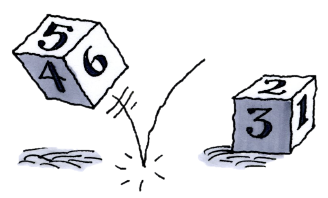### Home > INT2 > Chapter 3 > Lesson 3.1.3 > Problem3-30

3-30.

Use a probability area model, a tree diagram, or refer to the table you created for the Roll and Win situation (problem 3-6) that represents the sample space for the sum of the numbers when rolling two standard six-sided dice.

1. In a standard casino dice game the player wins on the first roll if he rolls a sum of $7$ or $11$. What is the probability of winning on the first roll?

$\frac{8}{36}$

2. The player loses on the first roll if he rolls a sum of $2, 3,$ or $12$. What is the probability of losing on the first roll?

Use the table.

3. If the player rolls any other sum, he continues to roll the dice until the first sum he rolled comes up again or until he rolls a sum of $7$ or $11$, whichever happens sooner. What is the probability that the game continues after the first roll?

What is the complement of the events in parts (a) and (b)?Use the table below to help you find the probabilities in parts (a) - (c).
Click the link at right for the full version of the eTool: Int2 3-30 HW eTool